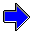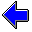Java Security Settings:
This web page employs Java, which requires specific security settings for correct operation.
If the applets on this page do not run correctly, consult the Virtual Chemistry Experiments FAQ
or the Physlet Physics web site for establishing the correct security settings.

# Kinetic Molecular Theory

## Basic Concepts

The gas laws developed by Boyle, Charles, and Gay-Lussac are based upon empirical observations and describe the behavior of a gas in macroscopic terms, that is, in terms of properties that a person can directly observe and experience. An alternative approach to understanding the behavior of a gas is to begin with the atomic theory, which states that all substances are composed of a large number of very small particles (molecules or atoms). In principle, the observable properties of gas (pressure, volume, temperature) are the consequence of the actions of the molecules making up the gas.

The Kinetic Molecular Theory of Gases begins with five postulates that describe the behavior of molecules in a gas. These postulates are based upon some simple, basic scientific notions, but they also involve some simplying assumptions. In reading a postulate, do two things. First, try to understand and appreciate the basic physical idea embodied in the postulate; this idea will ultimately be important in understanding the macroscopic properties of the gas in terms of the behavior the microscopic molecules making up the gas. Second, identify possible weakness or flaws in the postulates. Inaccurate predictions by a theory derive from flawed postulates used in the derivation of the theory.

### Postulates

1. A gas consists of a collection of small particles traveling in straight-line motion and obeying Newton's Laws.
2. The molecules in a gas occupy no volume (that is, they are points).
3. Collisions between molecules are perfectly elastic (that is, no energy is gained or lost during the collision).
4. There are no attractive or repulsive forces between the molecules.
5. The average kinetic energy of a molecule is 3kT/2. (T is the absolute temperature and k is the Boltzmann constant.)

### Behavior of Molecules in a Gas

To illustrate the significance of these postulates, consider the box containing a single molecule shown below. Start the animation and observe the molecule, represented by the blue ball, bouncing and traveling back and forth across the box. The collisions with the walls are perfectly elastic. Energy is neither gained nor lost from the collision. Because the walls do not move, the molecule's speed is unaffected by the collision. The graph plots the particle speed as a function of time. Observe that the speed has a constant value.

Now consider the box below in which several molecules exist. Start the animation and observe the blue molecule. Unlike the system above, this system has multiple molecules and thus collisions between molecules occur. Observe how the speed (and direction) of the molecule changes as a result of a collision. Thus the speed of a given molecule is not constant. From one collision to the next the molecule speeds up, slows down, speeds up, etc.

The graph shows how the speed changes with time. Observe that the speed is a constant in between collisions. At the moment of each collision there is an abrupt change in speed (and in direction).

Averaged over a long period of time, the average kinetic energy of a given molecule is 3kT/2. Similarly, the average kinetic energy of a large number of molecules at a particular instant in time is 3kT/2.

Maxwell DistributionKinetic Molecular Theory Home PageVirtual Chemistry Home Page

BasicConcepts.html version 1.3
© 2001-2014 David N. Blauch